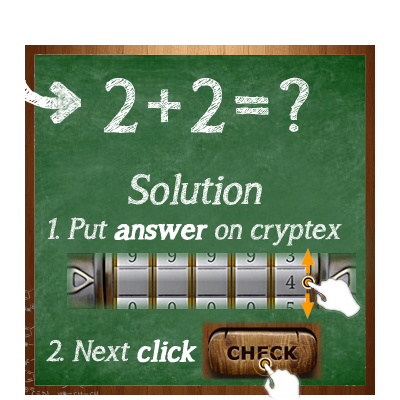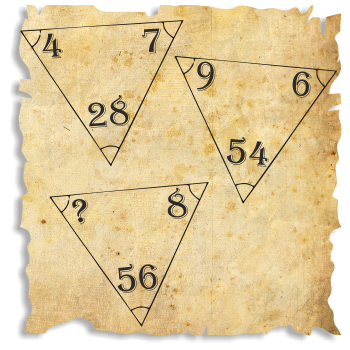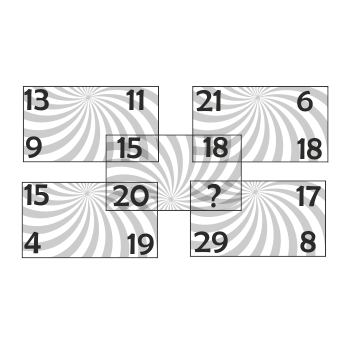Home | Android | IQ Test Cryptex all answers and hints# IQ Test Cryptex all answers and hints

IQ Test Cryptex all answers and hints

The game can be found on google play.

Level 1:

1. Hint 1   : here will be little help to solve riddle;
2. Hint 2  : here will be big help to solve riddle;
4. Image  :Level 2:

1. Hint 1   : numbers increase by a certain value;
2. Hint 2  : difference between numbers is 2;
4. Image  :
1. Hint 1   : water;
2. Hint 2  : chemical formula for water also known as HOH;
4. Image  :
1. Hint 1   : sum up the numbers in the columns;
2. Hint 2  : the sum of columns are increasing by 1;
4. Image  :
1. Hint 1   : use multiply;
2. Hint 2  : result is at the bottom of triangles;
4. Image  :Level 6:

1. Hint 1   : multiply diagonally;
2. Hint 2  : 15*4=60=12*5, 2*14=28=7*4, …;
4. Image  :
1. Hint 1   : order of the operations is important;
2. Hint 2  : division and multiplication is done before addition 2+2+2+2+((2*2):2);
4. Image  :
1. Hint 1   : increase the added number;
2. Hint 2  : 3+<u>2</u>=5, 5+<u>3</u>=8, 8+<u>4</u>=12 …;
4. Image  :
1. Hint 1   : compare the sum of the numbers in the groups;
2. Hint 2  : every group has the same result 1+2+3+4=<b>10</b> 4+2+3+1=<b>10</b>;
4. Image  :
1. Hint 1   : lines are important;
2. Hint 2  : count the lines :);
4. Image  :
1. Hint 1   : results on the bottom;
2. Hint 2  : make operations in columns;
4. Image  :
1. Hint 1   : Count squares! First square is made up of 5 squares.;
2. Hint 2  : Do you see the biggest suare? The biggest square consists of 9 smallest squares :). There are 3 kinds of squares: small, medium and big.;
4. Image  :
1. Hint 1   : A=8 B=8;
2. Hint 2  : C=5 D=7;
3. Answer: 31 & 26 or 26 & 31;
4. Image  :
1. Hint 1   : One of the four cardinal directions.;
2. Hint 2  : On Earth, we have 4 cardinal points: <b>N</b>ORTH, <b>E</b>AST, <b>W</b>EST, and …?;
4. Image  :
1. Hint 1   : decrease the number of less and less value;
2. Hint 2  : 143-<u>23</u>=120, 120-<u>21</u>=99, \uFFFD;
4. Image  :
1. Hint 1   : This is the Periodic Table. Do you remember this from chemistry class :)?;
2. Hint 2  : Oxygen in its molecular form.;
4. Image  :
1. Hint 1   : make some basic operations;
2. Hint 2  : sum up numbers and compare results;
4. Image  :
1. Hint 1   : Shapes are not the numbers!;
2. Hint 2  : Count the lines;
4. Image  :
1. Hint 1   : triangle = triangle = triangle;
2. Hint 2  : sum of each triangle is the same;
4. Image  :
1. Hint 1   : there are two separate sequences;
2. Hint 2  : 25, 24, 23, \uFFFD;
4. Image  :
1. Hint 1   : Symmetry is the key!;
2. Hint 2  : Focus on the right side of each symbol.;
4. Image  :
1. Hint 1   : find something common between numbers and letter;
2. Hint 2  : A=1 B=2 C=3 15=?;
4. Image  :
1. Hint 1   : first multiply then substract;
2. Hint 2  : 6*5-2, 7*2-9, …;
4. Image  :
1. Hint 1   : N=8;
2. Hint 2  : M=4,P=6;
4. Image  :
1. Hint 1   : find two separate sequences;
2. Hint 2  : -1&frac12;, +2&frac12;, -1&frac12;, +2&frac12;, …;
4. Image  :
1. Hint 1   : add rows;
2. Hint 2  : 350267+124623=?74890;
4. Image  :
1. Hint 1   : More figures, the greater the number is.;
2. Hint 2  : Number reflects the amount figures around. For example number \”3\” is included in 2 rectangles and a 1 circle.;
4. Image  :
1. Hint 1   : time passes faster and faste;
2. Hint 2  : 3:00 + <u>75min</u> = 4:15<br>4:15 + <u>150min</u> = 6:45<br>…;
4. Image  :
1. Hint 1   : start and finish at \”?\”(it’s about results);
2. Hint 2  : order of the operations is not important (result is between: 10 < ? < 15);
4. Image  :
1. Hint 1   : &#9632; = 6, &#9650; = 9;
2. Hint 2  : &#9632; = 6, &#9650; = 9, &#9679; = 3, &times; = 24;
4. Image  :
1. Hint 1   : sum up and then subtract;
2. Hint 2  : sum up vertical numbers then subtract numbers on sides;
4. Image  :
1. Hint 1   : make operations in the columns;
2. Hint 2  : the biggest number in a column is a result;
4. Image  :
1. Hint 1   : use symmetry with result;
2. Hint 2  : sum up the letters and see the result in the mirror;
4. Image  :
1. Hint 1   : basic operation is to multiply something;
2. Hint 2  : (5*3)-(1*9)=6;
4. Image  :Level 35:

1. Hint 1   : check difference between opposite letters;
2. Hint 2  : L-A= 11 (A=1, L=12);
4. Image  :
1. Hint 1   : sum up numbers, then try subtract something;
2. Hint 2  : 24-19=4;
4. Image  :
1. Hint 1   : different circles have different values;
2. Hint 2  : black circle = 7;
4. Image  :
1. Hint 1   : sum up first three numbers;
2. Hint 2  : 1+1+2=4, 1+2+4=7 …;
4. Image  :
1. Hint 1   : sum up the opposite letters;
2. Hint 2  : E(5)+H(8)=(13) …;
4. Image  :
1. Hint 1   : multiply digits, result is in the center;
2. Hint 2  : 2*4=8 6*9=54 (854);
4. Image  :
1. Hint 1   : sum up the numbers at the star corners;
2. Hint 2  : 28=82, 23=32, 31=13…;
4. Image  :
1. Hint 1   : move clockwise with intermission;
2. Hint 2  : A(B)C, B(CD)E …;
4. Image  :
1. Hint 1   : find similarity between letters and numbers;
2. Hint 2  : replace the letters with the numbers;
4. Image  :
1. Hint 1   : divide large square into smaller and make basic operations;
2. Hint 2  : sum of each 4 small squares is 15;
4. Image  :
1. Hint 1   : sum up numbers in the groups;
2. Hint 2  : every group has the same result;
4. Image  :
1. Hint 1   : number at the bottom is part of the top number;
2. Hint 2  : 588*0.25;
4. Image  :
1. Hint 1   : check the distance between the letters;
2. Hint 2  : distance between the letters equals 11;
4. Image  :
1. Hint 1   : sum up everything except the center;
2. Hint 2  : (36+41+49):3=42 …;
4. Image  :
1. Hint 1   : change letter to number;
2. Hint 2  : sum of 1 triangle = sum of 2 triangle (position of the letter in the alphabet);
4. Image  :
1. Hint 1   : Multiply vertically and horizontally;
2. Hint 2  : ((6*8)-(7*4)):4=5 …;
4. Image  :
1. Hint 1   : multiplication and subtraction;
2. Hint 2  : 8*4*9-…;
4. Image  :
1. Hint 1   : use subtraction and multiplication;
2. Hint 2  : subtract the edges and multiply the result by 2;
4. Image  :
1. Hint 1   : words have no meaning, count the lines;
2. Hint 2  : straight lines minus the curves;
4. Image  :
1. Hint 1   : replace the letters with the numbers;
2. Hint 2  : 4*4=16, 5*5=25, …;
4. Image  :
1. Hint 1   : add up the numbers on the sides;
2. Hint 2  : the number at the top equals &frac12 of the result;
4. Image  :
1. Hint 1   : difference between the number increases by more than a half;
2. Hint 2  : 1+1+1=3, 3+3+2=8, 8+8+3=19 . . .;
4. Image  :
1. Hint 1   : multiply two external numbers;
2. Hint 2  : 10&times;3=30:2=15, 8&times;7=56:2=28 …;
4. Image  :
1. Hint 1   : try to find some words;
2. Hint 2  : have you ever heard abort LOGO QUIZ game? by mateusz(funbox)klaczak(bubble)co.;
4. Image  :
1. Hint 1   : check the distance between the letters;
2. Hint 2  : multiply the distance between the letters by 2;
4. Image  :
1. Hint 1   : sum up digits not numbers;
2. Hint 2  : 1+3+1+1+9=15 …;
4. Image  :1.level 34 does not have to answer ? = 3
Example :
(6+4+2+3) – (3+2) = X
(5+1+9+3) – (9+3) = VI

so the formula for the question :
(9+2+8+?) – (8+?) = XI
and ? = 11
if that was true??

2.VINCENT MACIOLEK

LEVEL 49

D-4 SKIP 2 NUMBERS GOING CLOCKWISE
G-7
J-10
M-13
P-16
?-19

3.The 59th question is fucked up. The distance between G and Z is 19.

19×2=38

But how come the number given is 32??

4.Can you explain the solution to #51? That is the only one I still don’t get, even with the hints.

•(8*9*4)-(4+6+?)=271

5.silicone bracelet

Appreciate the recommendation. Will try it out.

6.Custom bracelets in NZ

You got a very excellent website, Glad I observed it through yahoo.

7.Cấp 25 ai giải thích giúp với

This site uses Akismet to reduce spam. Learn how your comment data is processed.